# Algebra II : Adding and Subtracting Logarithms

## Example Questions

### Example Question #11 : Adding And Subtracting Logarithms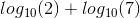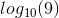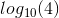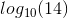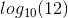Explanation:

When adding logarithms of the same base, all you have to do is multiply the numbers inside the function as shown below: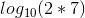### Example Question #12 : Adding And Subtracting Logarithms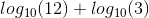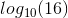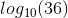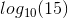Explanation:

When adding logarithms of the same base, all you have to do is multiply the numbers inside the function as shown below: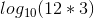### Example Question #13 : Adding And Subtracting Logarithms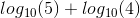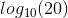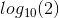Explanation:

When adding logarithms of the same base, all you have to do is multiply the numbers inside the function as shown below: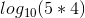### Example Question #14 : Adding And Subtracting Logarithms

Subtract the logarithms: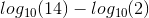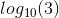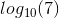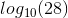Explanation:

When subtracting logarithms of the same base, all you have to do is divide the numbers inside the function as shown below: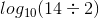### Example Question #15 : Adding And Subtracting Logarithms

Subtract the logarithms: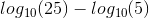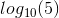Explanation:

When subtracting logarithms of the same base, all you have to do is divide the numbers inside the function as shown below: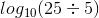### Example Question #16 : Adding And Subtracting Logarithms

Subtract the logarithms: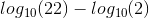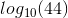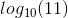Explanation:

When subtracting logarithms of the same base, all you have to do is divide the numbers inside the function as shown below: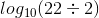### Example Question #17 : Adding And Subtracting Logarithms

Subtract the logarithms: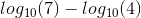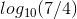Explanation:

When subtracting logarithms of the same base, all you have to do is divide the numbers inside the function as shown below: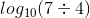### Example Question #18 : Adding And Subtracting Logarithms

Subtract the logarithms: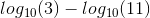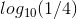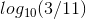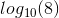Explanation:

When subtracting logarithms of the same base, all you have to do is divide the numbers inside the function as shown below: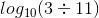### Example Question #19 : Adding And Subtracting Logarithms

Subtract the logarithms: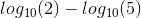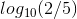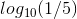Explanation:

When subtracting logarithms of the same base, all you have to do is divide the numbers inside the function as shown below: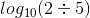### Example Question #20 : Adding And Subtracting Logarithms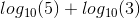Explanation:

When adding logarithms of the same base, all you have to do is multiply the numbers inside the function as shown below: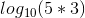### All Algebra II Resources